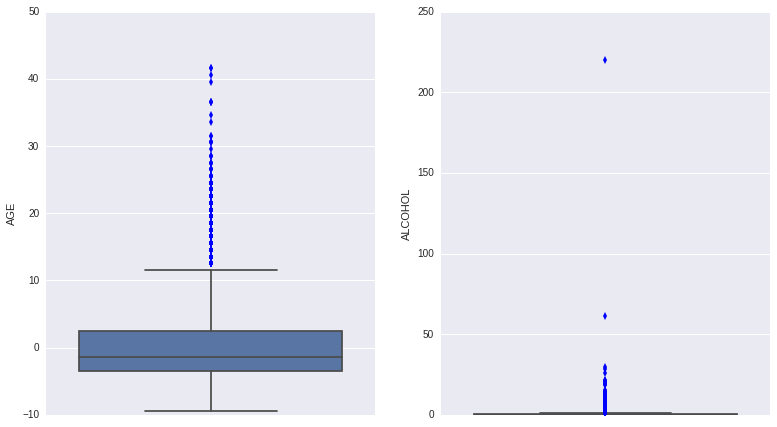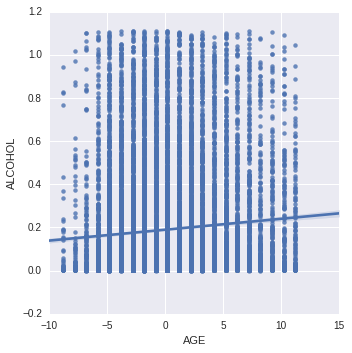# samucoder

Samuel M.H. 's technological blog

Notebook

# Test a Basic Linear Regression Model¶

Author: Samuel M.H. <samuel.mh@gmail.com> Date: 28-02-2016

## Instructions

This week's assignment asks you to test a basic linear regression model for the association between your primary explanatory variable and a response variable, and to create a blog entry describing your results.

### Data preparation for this assignment:

1. If your explanatory variable is categorical with more than two categories, you will need to collapse it down to two categories, or subset your data to select observations from 2 categories (next week you'll learn how to analyze categorical explanatory variable with more than 2 categories).

2. If your response variable is categorical, you will need to identify a quantitative variable in the data set that you can use as a response variable for this assignment. Variables with response scales with 4-5 values that represent a change in magnitude (for example, "strongly disagree to strongly agree", "never to often") can be considered quantitative for the assignment.

### The assignment:

1. If you have a categorical explanatory variable, make sure one of your categories is coded "0" and generate a frequency table for this variable to check your coding. If you have a quantitative explanatory variable, center it so that the mean = 0 (or really close to 0) by subtracting the mean, and then calculate the mean to check your centering.

2. Test a linear regression model and summarize the results in a couple of sentences. Make sure to include statistical results (regression coefficients and p-values) in your summary.

## What to submit

Create a blog entry where you 1) post your program and output, and 2) post a frequency table for your (recoded) categorical explanatory variable or report the mean for your centered explanatory variable. 3) Write a few sentences describing the results of your linear regression analysis.

## Intro

In this exercise I will test the association between the age at which a person gets married for the first time and the alcohol they consumed during last year.

## Dataset

### Variables

• Explanatory: S1Q4A, age at first marriage.
• Quantitative: 14-94 years, 31794 samples
• Unknown values (99) and never married people are discarded.
• Response: ETOTLCA2, average daily volume of ethanol consumed in past year, from all types of alcoholic beverages combined.
• Quantitative: 0.0003-219.955 oz. ethanol/day
• Unkwnown values are discarded observations. Could be mapped to the mean, but don't add much.
In :
%matplotlib inline

import numpy as np
import pandas as pd
import statsmodels.formula.api as smf
import seaborn as sns
import matplotlib.pyplot as plt
#import scipy


## Data

In :
# Load data

# Custom dataframe
df = pd.DataFrame()
df['AGE'] = data['S1Q4A'].replace(' ',np.NaN).replace('99',np.NaN).astype(float)
df['ALCOHOL'] = data['ETOTLCA2'].replace(' ',np.NaN).astype(float)
df = df.dropna()

# Center the explanatory variable
print('Original')
print(df.describe())
df['AGE'] = df['AGE']- df['AGE'].mean()
print('\n\nCentered AGE')
print(df.describe())

Original
AGE       ALCOHOL
count  19277.000000  19277.000000
mean      23.456710      0.494287
std        5.161488      2.034222
min       14.000000      0.000300
25%       20.000000      0.014700
50%       22.000000      0.094500
75%       26.000000      0.453100
max       65.000000    219.955500

Centered AGE
AGE       ALCOHOL
count  1.927700e+04  19277.000000
mean   3.066720e-16      0.494287
std    5.161488e+00      2.034222
min   -9.456710e+00      0.000300
25%   -3.456710e+00      0.014700
50%   -1.456710e+00      0.094500
75%    2.543290e+00      0.453100
max    4.154329e+01    219.955500



We see the final population contains 19277 samples and the AGE variable has been centered, zero mean.

### Outliers

In order to improve the generalization an obtain better results, I will perform an analysis of the outliers.

These are the boxplots:

In :
# Boxplots
fig, (ax1,ax2)= plt.subplots(1,2)
bp_age = sns.boxplot(x='AGE',data=df, whis=1.5, orient='v', ax=ax1)
bp_alcohol = sns.boxplot(x='ALCOHOL',data=df, whis=1.5, orient='v', ax=(ax2))
fig.show()It is possible to see that there are several outliers in the AGE variable as well as in the ALCOHOL one. The procedure will be calculating the interquartile range and discarding the observations greater than 1.5 these values (the blue points).

Values $v$ that satisfy any of these properties are discarded: $$v_{max} > Q_3 + 1.5 (Q_3-Q_1)\\ v_{min} < Q_1 - 1.5 (Q_3-Q_1)$$

In :
#Calculate minimum and maximum values for outliers
def outlier_limits(data, whis=1.5):
q3 = np.percentile(data, 75)
q1 = np.percentile(data, 25)
iqr = q3-q1
return(
q1-(whis*iqr),
q3+(whis*iqr),
)

In :
# Outlier limits
print('Allowed ranges')
print('{0} < AGE < {1}'.format(*outlier_limits(df['AGE'])))
print('{0} < ALCOHOL < {1}'.format(*outlier_limits(df['ALCOHOL'])))

Allowed ranges
-12.456710069 < AGE < 11.543289931
-0.6429 < ALCOHOL < 1.1107


In :
age_lower,age_upper= outlier_limits(df['AGE'])
alc_lower,alc_upper= outlier_limits(df['ALCOHOL'])

df = df[
(df['AGE']>age_lower) &
(df['AGE']<age_upper) &
(df['ALCOHOL']>alc_lower) &
(df['ALCOHOL']<alc_upper)
]
df['AGE'] = df['AGE']- df['AGE'].mean()
df.describe()

Out:
AGE ALCOHOL
count 1.645100e+04 16451.000000
mean 2.684349e-16 0.189769
std 4.055066e+00 0.260015
min -8.798128e+00 0.000300
25% -2.798128e+00 0.011700
50% -7.981278e-01 0.061600
75% 2.201872e+00 0.271500
max 1.120187e+01 1.110200

It is possible to see that all the values are in range, outliers have been removed and the population is formed by 16451 samples.

## Regression model

In :
model = smf.ols(formula='ALCOHOL~AGE',data=df).fit()
print model.summary()

                            OLS Regression Results
==============================================================================
Dep. Variable:                ALCOHOL   R-squared:                       0.006
Method:                 Least Squares   F-statistic:                     102.9
Date:                Sat, 27 Feb 2016   Prob (F-statistic):           4.18e-24
Time:                        22:45:48   Log-Likelihood:                -1131.4
No. Observations:               16451   AIC:                             2267.
Df Residuals:                   16449   BIC:                             2282.
Df Model:                           1
Covariance Type:            nonrobust
==============================================================================
coef    std err          t      P>|t|      [95.0% Conf. Int.]
------------------------------------------------------------------------------
Intercept      0.1898      0.002     93.900      0.000         0.186     0.194
AGE            0.0051      0.000     10.143      0.000         0.004     0.006
==============================================================================
Omnibus:                     4704.441   Durbin-Watson:                   2.013
Prob(Omnibus):                  0.000   Jarque-Bera (JB):            10471.995
Skew:                           1.674   Prob(JB):                         0.00
Kurtosis:                       5.016   Cond. No.                         4.05
==============================================================================

Warnings:
 Standard Errors assume that the covariance matrix of the errors is correctly specified.



## Summary

The p-value (nearly zero) is lower than 0.05. Therefore, the null hypothesis can be rejected as there is statistical evidence of the association between the variables.

The R-squared value is nearly 0, so, almost no variance can be explained by the AGE and the predictive equation $$\hat{ALCOHOL}=AGE*0.0051+0.1898$$ is useless. There is no correlation between variables.

This is a scatter plot with the fitted line.

In :
sns.lmplot(x='AGE', y='ALCOHOL', data=df)

Out:
<seaborn.axisgrid.FacetGrid at 0x7f6aa5c21790>It is possible to see the plot does not comply with the homoscedasticity assumption for the linear regression model.

The linear model cannot decribe the relationship between the age at which one gets married and the alcohol they drank during last year, but, the relationship has statistical significance (it did not happen by chance).

#### 1 comment:

1.How did you run the regression model without getting an error? When I typed in smf.ols, I got this error: ValueError: For numerical factors, num_columns must be an int